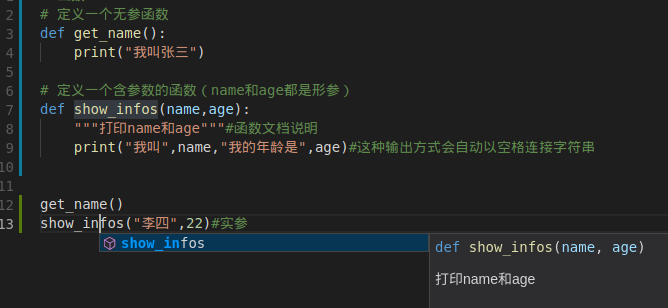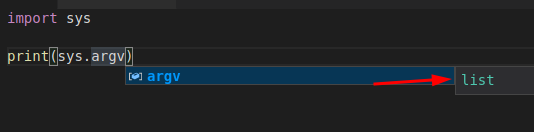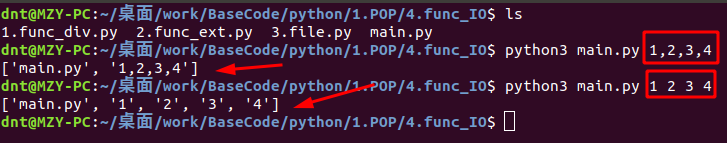# Python3 与 C# 基础语法对比（Function专栏）

## 1.Python函数定义¶

### 1.1.定义一个无参函数¶

In :
# 空函数定义
def method():
pass #相当于占位符，ifelse等等里面都是可以出现的

method()

In :
# 定义一个无参函数
def get_name():
print("我叫张三")

In :
# 调用一个无参函数
get_name()


我叫张三


### 1.2.定义一个含参函数¶In :
# 定义一个含参数的函数（name和age都是形参）
def show_infos(name,age):
"""打印name和age"""#函数文档说明
print("我叫",name,"我的年龄是",age)#这种输出方式会自动以空格连接字符串

In :
# 调用一个含参数的函数
show_infos("李四",22)#实参


我叫 李四 我的年龄是 22

In :
# 定义一个含默认参数(缺省参数)的函数（默认参数必须指向不变对象）
# 比如你设置一个list，改变了内容，则下次调用时，默认参数(缺省参数)的内容就变了，不再是函数定义时的值了
def default_param(name,age=23):
"""age默认为23"""
print("我叫",name,"我的年龄是",age)#这种输出方式会自动以空格连接字符串

In :
# 调用一个默认参数(缺省参数)的函数
default_param("张三")


我叫 张三 我的年龄是 23


### 1.3.定义有返回值的函数¶

In :
# 定义有返回值的函数
def div_have_return(a,b):
"""计算a+b，返回计算结果"""#函数文档说明
return a+b

In :
# 调用有返回值的函数
result=div_have_return(1,2)
print("计算结果为",result)


计算结果为 3

In :
# 定义含有多个返回值的函数（利用了元组）
def div_have_returns(a,b):
"""计算a+b的和，计算a-b，并返回两个结果"""
return (a+b),(a-b)

In :
# 调用含有多个返回值的函数
sum,dvalue=div_have_returns(1,2)
print("sum:",sum,"D-value:",dvalue)


sum: 3 D-value: -1

In :
# 测试一下～返回多个值其实是利用了元组
# 多返回值只是一种假象，Python函数返回的仍然是单一值～元组
test=div_have_returns(1,2)
print(test)


(3, -1)

In :
# 定义函数时，需要确定函数名和参数个数
# 如果有必要，可以先对参数的数据类型做检查
# 函数体内部可以用return随时返回函数结果
# 函数执行完毕也没有return语句时，自动return None
# 函数可以同时返回多个值，但其实就是一个tuple

In :
# 扩展：使用list实现
# 定义含有多个返回值的函数（利用了元组）
def div_have_returns(a,b):
"""计算a+b的和，计算a-b，并返回两个结果"""
return [a+b,a-b]

In :
# 调用含有多个返回值的函数
div_have_returns(1,2)

Out:
[3, -1]

## 2.Python函数传参系列¶

### 2.1.引用传递（通过元组、列表实现）¶

In :
######## 通过元组、列表实现 ########
def default_some_params(nums):
"""借助Tuple和list"""
sum=0
for item in nums:
sum+=item
return sum

In :
# 元组传入
default_some_params((1,2,3,4,5))

Out:
15
In :
# 列表传入
default_some_params(list(range(1,6)))

Out:
15

### 2.2.可变参数¶

In :
######## 定义一个可变参数的函数(名字一般都是*args) ########
# 定义可变参数和定义一个list或tuple参数相比，仅仅在参数前面加了一个*号。
# 在函数内部，接收到的参数是一个tuple。调用该函数时，可以传入任意个参数（包括0个参数）
def default_params(*args):
"""定义一个可变参数,用来求所有参数的总和"""
sum=0
for item in args:
sum+=item
return sum

In :
# 调用一个可变参数的函数
default_params(1,2,3,4,5)

Out:
15
In :
# 传一个list或者Tuple（参数前面加*）
# 传一个元组
test_tuple=(1,2,3,4,5)
default_params(*test_tuple)

Out:
15
In :
# range(1,6) ==> [1,6) ==> 1,2,3,4,5
test_list=list(range(1,6))
default_params(*test_list)

Out:
15### 2.3.关键字参数¶

In :
######## 调用含关键字参数的函数 ########
# 可变参数允许你传入0个或任意个参数，这些可变参数在函数调用时自动组装为一个tuple
# 关键字参数允许你传入0个或任意个含key-value的参数，自动组装为一个dict
def default_kv_params(name,age=23,**kv):
"""输出输出用户传参"""
print("我叫",name,"我的年龄是",age,"dict:",kv)

In :
# 调用含关键字参数的函数
default_kv_params("dnt",web="www.baidu.com",qq=110)


我叫 dnt 我的年龄是 23 dict: {'web': 'www.baidu.com', 'qq': 110}


In :
#### 同时定义可变参数+关键字参数 ####
def default_god_params(name,age=23,*args,**kv):
"""同时有可变参数+关键字参数的使用方法"""
print("我叫",name,"我的年龄是",age,"list:",args,"dict:",kv)

In :
# 调用可变参数+关键字参数
# 有名字给kv,没名字给args
default_god_params("dnt",24,1,2,3,4,5,web="www.baidu.com",qq=110)


我叫 dnt 我的年龄是 24 list: (1, 2, 3, 4, 5) dict: {'web': 'www.baidu.com', 'qq': 110}

In :
#只调用可变参数
default_god_params("dnt",24,1,2,3,4,5)


我叫 dnt 我的年龄是 24 list: (1, 2, 3, 4, 5) dict: {}

In :
# 只调用关键字参数
default_god_params("dnt",web="www.baidu.com",qq=110)


我叫 dnt 我的年龄是 23 list: () dict: {'web': 'www.baidu.com', 'qq': 110}

In :
#### 传元组和字典 ####
test_tuple=(1,2,3,4,5)
test_dict={"web":"www.baidu.com","qq":110}

default_god_params(*test_tuple,**test_dict)


我叫 1 我的年龄是 2 list: (3, 4, 5) dict: {'web': 'www.baidu.com', 'qq': 110}


## 3.CSharp函数基础¶

C#基础语法大家都很熟了，我贴一个注释的Code即可（Code在线

VSCode里面如果也想像VS一样，///就生成注释 ==》请安装函数文档注释：XML Documentation Comments

using System;
using System.Collections.Generic;

namespace _6func
{
class Program
{
static void Main(string[] args)
{
// 交换两数新方式
int x = 3, y = 5;
(x, y) = (y, x);
System.Console.WriteLine("{0},{1}", x, y);

#region Base
// # 定义一个空函数：
Method();
// # 定义一个无参函数
GetName();
// # 定义一个含参函数
ShowInfos("李四", 22);
// # 定义一个含默认参数(缺省参数)的函数
DefaultParam("张三");
// # 定义有返回值的函数
int result = DivHaveReturn(1, 2);
Console.WriteLine($"计算结果为{result}"); #endregion // # 定义含有多个返回值的函数（利用了元组） var (sum, dvalue) = DivHaveReturns(1, 2); Console.WriteLine($"sum:{sum},D-value:{dvalue}");

// 传多个参数系列：
// 引用传递（通过元组、列表实现）：扩展有可变类型和不可变类型作为形参的对比
var list = new List<int>() { 1, 2, 3, 4, 5 };
Console.WriteLine(DefaultSomeParams(list));
Console.WriteLine(list.Count);//这就是引用传递的证明

// # 定义一个可变参数的函数(参数名字一般都是*args)
Console.WriteLine(DefaultParams(1, 2, 3, 4, 5));

// # 定义含关键字参数的函数 直接传Dict
}

#region base
/// <summary>
/// 定义一个空函数
/// </summary>
private static void Method()
{

}
/// <summary>
/// 定义一个无参函数
/// </summary>
// private static void GetName()
// {
//     Console.WriteLine("你好");
// }
//简写
private static void GetName() => Console.WriteLine("你好");

/// <summary>
/// 定义一个含参数的函数
/// </summary>
/// <param name="name">名字</param>
/// <param name="age">年龄</param>
// private static void ShowInfos(string name, int age)
// {
//     Console.WriteLine($"我叫{name} 我的年龄是{age}"); // } //简写 private static void ShowInfos(string name, int age) => Console.WriteLine($"我叫{name} 我的年龄是{age}");

/// <summary>
/// 定义一个含缺省参数的函数
/// </summary>
/// <param name="name">名字</param>
/// <param name="age">年龄默认23</param>
// private static void DefaultParam(string name, int age = 23)
// {
//     Console.WriteLine($"我叫{name} 我的年龄是{age}"); // } //简写 private static void DefaultParam(string name, int age = 23) => Console.WriteLine($"我叫{name} 我的年龄是{age}");

/// <summary>
/// 定义一个有返回值的函数（计算a+b，返回计算结果）
/// </summary>
/// <param name="a">num1</param>
/// <param name="b">num2</param>
// private static int DivHaveReturn(int a, int b)
// {
//     return a + b;
// }
//简写
private static int DivHaveReturn(int a, int b) => a + b;
#endregion

/// <summary>
/// 定义含有多个返回值的函数（利用了元组）
/// 计算a+b的和，计算a-b，并返回两个结果
/// </summary>
/// <param name="a"></param>
/// <param name="b"></param>
/// <returns></returns>
// private static (int sum,int dValue) DivHaveReturns(int a, int b)
// {
//     return ((a+b),(a-b));
// }
//简写
private static (int sum, int dValue) DivHaveReturns(int a, int b) => ((a + b), (a - b));

#region 传入多个参数系列
/// <summary>
/// 利用列表实现,引用传递之类的C#还有ref和out，这边就不说了
/// </summary>
/// <param name="nums"></param>
/// <returns></returns>
private static int DefaultSomeParams(List<int> numList)
{
int sum = 0;
foreach (var item in numList)
{
sum += item;
}
numList.Clear();
return sum;
}
/// <summary>
/// 定义一个可变参数的函数
/// </summary>
/// <param name="args"></param>
/// <returns></returns>
private static int DefaultParams(params int[] args)
{
int sum = 0;
foreach (var item in args)
{
sum += item;
}
return sum;
}
#endregion
}
}


## 4.函数扩展¶

### 4.1.Python递归函数¶

In :
# 函数递归调用其实就是自己调用自己，关键点只要考虑什么时候跳出即可(没有跳出就是死循环)
# 阶乘案例 n!
def factorial(n):
if n==1:
return n #跳出
elif n>1:
return n*factorial(n-1) #规律公式

In :
print(factorial(4))
print(factorial(-4))


24
None


In :
# 利用切片操作，实现一个trim()函数，去除字符串首尾的空格
# 跳出点==> 第一个字符和最后一个字符不是空格
def my_trim(input_str):
if input_str != " " and input_str[-1] != " ":
return input_str
elif input_str==" ":
return my_trim(input_str[1:])#从第二个到最后一个字符
elif input_str[-1]==" ":
return my_trim(input_str[:-1])#从第一个到倒数第二个(end_index取不到)

In :
print(my_trim("我去  "))
print(my_trim("  我去"))
print(my_trim("  我去  "))


我去



### 4.2.CSharp递归¶

C#的递归我就引用一下以前的（Code

using System;

namespace _10.递归案例
{
class Program
{
//一列数的规则如下: 1、1、2、3、5、8、13、21、34...... 求第30位数是多少， 用递归算法实现
static void Main(string[] args)
{
//1     2       3       4       5           n
//1     1      1+1     2+1     3+2   Fn(n-2)+Fn(n-1)
Console.WriteLine(GetNum(30));
Console.ReadKey();
}
/// <summary>
/// 递归就这么理解，先找能打破重复的条件，然后就不断的重复去吧
/// </summary>
/// <param name="n"></param>
/// <returns></returns>
private static int GetNum(int n)
{
if (n == 1 || n == 2)
{
return 1;
}
else
{
return GetNum(n - 1) + GetNum(n - 2);
}

}
}
}


### 4.3.匿名函数¶

C#上面Code中的简写都是用匿名函数的方式，可以参考。

In :
# Python对匿名函数的支持有限，只有一些简单的情况下可以使用匿名函数
# lambda 参数: 表达式
# 来个简单求和案例:
sum=lambda a,b: a+b

In :
sum(1,2) #调用一下看看(有点js的感觉,函数可以直接赋值给变量,然后直接用)

Out:
3

In :
# 来个实际案例,还记得list的排序吗?
# 这次按照指定key排序(有点 SQL 里面 order by 的感觉)
data_list=[
{"name":"a张三","age":21},
{"name":"b李四","age":23},
{"name":"a王五","age":22}]

In :
# 按照age排序
data_list.sort(key=lambda x:x["age"])
print(data_list)

# 按照name排序
data_list.sort(key=lambda x:x["name"])
print(data_list)


[{'name': 'a张三', 'age': 21}, {'name': 'a王五', 'age': 22}, {'name': 'b李四', 'age': 23}]
[{'name': 'a张三', 'age': 21}, {'name': 'a王五', 'age': 22}, {'name': 'b李四', 'age': 23}]

In :
# 可以看看帮助文档
help(data_list.sort)


Help on built-in function sort:

sort(...) method of builtins.list instance
L.sort(key=None, reverse=False) -> None -- stable sort *IN PLACE*


In :
# 当函数的参数传递(有时候需要传一个匿名方法去函数中处理某些事情)
def sum(a,b,func):
return func(a,b)

In :
sum(1,2,lambda x,y: x+y)

Out:
3

### 4.4.全局变量和局部变量¶

In :
# 有人可能会说，这个就太简单了，没啥好说的，(⊙o⊙)… Python还真需要说说
a=100
b=[1,2]

def set_num(num):
num+=num

set_num(a)
set_num(b)

In :
# 发现全局变量a木有被修改，而b修改了
print(a)
print(b)


100
[1, 2, 1, 2]


In :
# 还是来个简单案例说明下吧～
a=100

def set_num(num):
a=200

set_num(a)
print(a)


100


In :
# a=200，python解释器就认为你定义了一个和a这个全局变量名字相同的局部变量，那怎么用呢？global来声明一下即可
a=100

def set_num(num):
global a
a=200

set_num(a)
print(a)


200


### 4.5.可变类型和不可变类型¶

Python分为：可变类型：list，dict,set等等 和 不可变类型：int，str，tuple，float等等

In :
a="mmd"

In :
a

Out:
'm'
In :
# 没法修改
a='d'


---------------------------------------------------------------------------
TypeError                                 Traceback (most recent call last)
<ipython-input-3-f2c76b4f2b09> in <module>()
1 # 没法修改
----> 2a='d'

TypeError: 'str' object does not support item assignment
In :
a=['m','m','d']
a

Out:
'm'
In :
a='n'
a

Out:
['n', 'm', 'd']

In :
i=1
id(i)

Out:
94490537630240
In :
# 地址改变了
i+=1
id(i)

Out:
94490537630272
In :
# 地址又变了
i=i+1
id(i)

Out:
94490537630304

In :
j=1
id(j) # 有没有发现，跟一开始i的内存地址一样了

Out:
94490537630240

In :
# 更直观的来几个案例
a=1
b=1
c=1

In :
# 不仅仅都一样，还和所有等于1的变量地址都相同
# 这下不怀疑了吧
print(id(a))
print(id(b))
print(id(c))


94490537630240
94490537630240
94490537630240


In :
f1=1.2
id(f1)

Out:
139755722935032
In :
# 地址变了，说明float也是不可变类型
f1+=1.0
id(f1)

Out:
139755722934936

In :
f2=1.2
f3=1.2
print(id(f2))
print(id(f3))


139755722935224
139755722935128


In :
list1=[12,11,1]
id(list1)

Out:
139755687637576
In :
# 改完还是自己原来的地址～说明是可变类型
list1.append(0)
id(list1)

Out:
139755687637576

In :
# 当存在多个值相同的不可变类型变量时，并不是指向同一个地址
list2=[12,11,1]
list3=[12,11,1]
print(id(list2))
print(id(list3))


139755687065160
139755687093704


In :
print(id(list2))
print(id(list3))
list3=list2
print(id(list3)) # 现在list3的地址和list2地址一样了


139755687065160
139755687093704
139755687065160

In :
# 注意这种情况，如果对其中一个list修改，那么另一个也就跟着被修改了
# 任意一个List进行修改，都会影响另外一个List的值
list2.append(0)
print(list2)
print(list3)
print(id(list2))
print(id(list3))


[12, 11, 1, 0]
[12, 11, 1, 0]
139755687065160
139755687065160


### 4.6.验证扩展系列¶

1.之前很多资料说python3的匿名函数里不能调用print函数,自己测试下：

In :
# 之前很多资料说py3匿名函数里不能调用print函数,自己测试下
# 定义一个无参匿名函数
printf=lambda:print("I Love You")

In :
printf()


I Love You


2.可变类型与不可变类型的变量分别作为函数参数的区别

Python中函数参数都是引用传递

In :
# 可变类型与不可变类型的变量分别作为函数参数的区别
def default_some_params(nums):
nums+=nums

In :
test_num=1
default_some_params(test_num)
test_num

Out:
1
In :
test_list=list(range(1,6))
default_some_params(test_list)
test_list

Out:
[1, 2, 3, 4, 5, 1, 2, 3, 4, 5]

3.函数名能不能重复的问题

In :
# 函数名能不能重复的问题（能不能重载：具有不同的参数的类型或参数的个数）
def test():
pass

def test(a,b):
return a+b

In :
test(1,2)

Out:
3
In :
test() #前一个直接被后一个覆盖掉了


---------------------------------------------------------------------------
TypeError                                 Traceback (most recent call last)
<ipython-input-29-023d21616394> in <module>()
----> 1test() #前一个直接被后一个覆盖掉了

TypeError: test() missing 2 required positional arguments: 'a' and 'b'

4.这次说说两个有趣的扩展

In :
# 交换两数～元组的方式
a=1
b=2
a,b=b,a # 写全：(a,b)=(b,a)
print(a)
print(b)


2
1


C#的再回顾一下

int x = 1, y = 2;
(x, y) = (y, x);
Console.WriteLine("x： " + x + "  y： " + x);


5.eval（和js里面的eval差不多）：不太建议使用

In :
# 2.eval（和js里面的eval差不多）：
input_str=input("请输入：")
print(input_str)
eval(input_str)


请输入：print("厉害")
print("厉害")


posted @ 2018-06-13 13:22  鲲逸鹏  阅读(2868)  评论(17编辑  收藏  举报